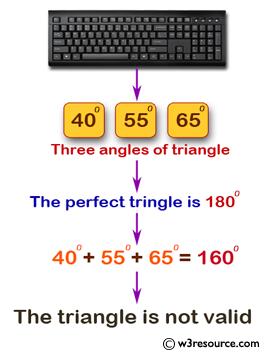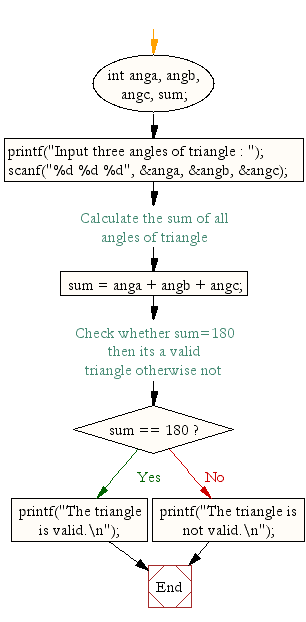﻿ C : Check whether a triangle can be formed by given value# C Exercises: Check whether a triangle can be formed by given value

## C Conditional Statement: Exercise-15 with Solution

Write a C program to check whether a triangle can be formed by the given value for the angles.

Pictorial Presentation:Sample Solution:

C Code:

``````#include <stdio.h>
void main()
{
int anga, angb, angc, sum; //are three angles of a triangle

printf("Input three angles of triangle : ");
scanf("%d %d %d", &anga, &angb, &angc);

/* Calculate the sum of all angles of triangle */
sum = anga + angb + angc;

/* Check whether sum=180 then its a valid triangle otherwise not */
if(sum == 180)
{
printf("The triangle is valid.\n");
}
else
{
printf("The triangle is not valid.\n");
}
}
```
```

Sample Output:

```Input three angles of triangle : 40 55 65
The triangle is not valid.
```

Flowchart:C Programming Code Editor:

Improve this sample solution and post your code through Disqus.

What is the difficulty level of this exercise?

Test your Programming skills with w3resource's quiz.

﻿

## C Programming: Tips of the Day

Why do C and C++ compilers allow array lengths in function signatures when they're never enforced?

It is a quirk of the syntax for passing arrays to functions.

Actually it is not possible to pass an array in C. If you write syntax that looks like it should pass the array, what actually happens is that a pointer to the first element of the array is passed instead.

Since the pointer does not include any length information, the contents of your [] in the function formal parameter list are actually ignored.

Ref : https://bit.ly/3fhlvdH